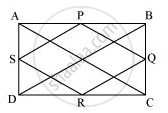# ABCD is a rectangle and P, Q, R and S are mid-points of the sides AB, BC, CD and DA respectively. Show that the quadrilateral PQRS is a rhombus. - Mathematics

ABCD is a rectangle and P, Q, R and S are mid-points of the sides AB, BC, CD and DA respectively. Show that the quadrilateral PQRS is a rhombus.

#### SolutionLet us join AC and BD.

In ΔABC,

P and Q are the mid-points of AB and BC respectively.

∴ PQ || AC and PQ = 1/2AC (Mid-point theorem) ... (1)

SR || AC and SR = 1/2AC (Mid-point theorem) ... (2)

Clearly, PQ || SR and PQ = SR

Since in quadrilateral PQRS, one pair of opposite sides is equal and parallel to

each other, it is a parallelogram.

∴ PS || QR and PS = QR (Opposite sides of parallelogram)... (3)

In ΔBCD, Q and R are the mid-points of side BC and CD respectively.

∴ QR || BD and QR =1/2BD (Mid-point theorem) ... (4)

However, the diagonals of a rectangle are equal.

∴ AC = BD …(5)

By using equation (1), (2), (3), (4), and (5), we obtain

PQ = QR = SR = PS

Therefore, PQRS is a rhombus.

Concept: The Mid-point Theorem
Is there an error in this question or solution?

#### APPEARS IN

NCERT Class 9 Maths